# Maharashtra Board Class 12 Economics Important Questions Chapter 4 Supply Analysis

Balbharti Maharashtra State Board Class 12 Economics Important Questions Chapter 4 Supply Analysis Important Questions and Answers.

## Maharashtra State Board 12th Economics Important Questions Chapter 4 Supply Analysis

1.[A] Choose the correct option.

Question 1.
Net addition made to the total revenue by selling an extra unit of a commodity is
(a) total revenue
(b) marginal revenue
(c) average revenue
(d) marginal cost
(b) marginal revenue

Question 2.
Determinants of supply are …………………..
(a) cost of production
(b) short period
(c) constant price
(d) local market
(a) cost of productionQuestion 3.
Statement related to reservation price are
(a) maximum price
(b) constant price
(c) same for all seller
(d) differ from seller to seller
(d) differ from seller to seller

Question 4.
Exceptions to the law of supply are
(a) need for cash
(b) upward sloping supply curve
(c) factory products
(d) future expectation of high price
(a) need for cash

Question 5.
Average Revenue is obtained when .
(a) price is multiplied with quantity
(b) last unit is sold
(c) total revenue is divided by quantity sold
(d) all of these
(c) total revenue is divided by quantity sold

Question 6.
Increase in supply is shown by
(a) price change only
(b) movement on the same supply curve
(c) shift of supply curve to the left
(d) shift of supply curve to the right
(d) shift of supply curve to the right

(B) Complete Correlation

1. Rare goods : Exception to Law of Supply :: Assumption of Law of Supply : ……………….
2. ………………. : Individual supply :: Macro concept: Total supply
3. Marginal Revenue : TRn – TRn-1 :: ……………….: TR/TQ
4. Single seller : Individual Supply :: All sellers : ……………….
5. Movement on same supply curve : ……………….:: Shift of supply curve: Change in supply.

1. No change in Government Policy
2. Micro concept
3. Average Revenue
4. Market Supply
5. Variation in supply

(C) Give economic terms.

1. Creation of utility.
2. Outcome of the process of production.
3. Total quantity available with the producer for sale at time.
4. Sum total of individual supply.
5. Cost incurred on fixed factors like land, machinery etc.
6. Minimum price below which a seller will not sell a single unit.
7. Expansion and contraction of supply due to change in price.
8. Rise in supply due to fall in taxes.
9. Fall in supply due to fall in taxes.
10. Cost incurred on variable factor like raw material.
11. Net revenue earned by selling an additional unit.

1. Production
2. Output
3. Stock
4. Market Supply
5. Fixed Cost
6. Reservation Price
7. Variation in Supply
8. Increase in Supply
9. Decrease in Supply
10. Variable Cost
11. Marginal Revenue(D) Find the odd word out:

(1) Land, Labour, Capital, Rent.
(2) Rent, Wages, Profit, Capital.
(3) AC, TC, TFC, AR.
(4) Samuelson, Marshall, Robbins, Abdul Kalam.
(5) [ncrease in supply, Decrease in supply, Extension in supply, Market supply.

1. Rent
2. Capital
3. AR
4. Abdul Kalam
5. Market supply

(E) Complete the following statements.

1. Production consists of various processes through which ………………
2. Stock determines ………………
3. Stock and supply are same in case of ………………
4. Sum total of individual supply is called ………………
5. Graphical representation of market supply schedule is called ………………
6. When quantity supplied rises due to an increase in price of a commodity, it is called ………………
7. When more quantity is supplied at the same price of a commodity, it is called ………………
8. Per unit cost of production is called ………………
9. Total expenditure incurred by a firm on factors of production for the production of goods and services is called ………………
10. An addition made to TR by selling one additional unit of a commodity is called ………………

1. utility is created
2. potential supply
3. perishable goods
4. market supply
5. market supply curve
6. extension or expansion in supply
7. increases in supply
8. Average Cost
9. Total Cost
10. Marginal Revenue

(F) Choose the wrong pair:

I.

 Group ‘A’ Group ‘B’ 1. Total Output Sum of quantity produced 2. Stock Reservation Price 3. Supply Quantity offered for sale

Wrong pair : Stock – Reservation price

II.

 Group ‘A’ Group ‘B’ 1. Extension in supply More supply at higher price 2. Contraction in supply Less supply at lower price 3. Increases in supply Part of variation in supply

Wrong pair : Increase in supply – Part of variation in supply

III.

 Group ‘A’ Group ‘B’ 1. Total Revenue Price x Quantity 2. Total Cost TFC + TVC 3. Average Cost TR x TQ

Wrong pair : Average Cost – TR x TQ(G) Choose the correct pair:

I.

 Group ‘A’ Group ‘B’ 1. Increase in supply Technology up gradation 2. Exception to law of supply More supply at higher price 3. Determination of law of supply Perishable Goods

Correct pair : Increases in supply – Technology up gradation

II.

 Group ‘A’ Group ‘B’ 1. Individual supply Potential supply 2. Determinants of law of supply Infrastructural facilities 3. Assumption of the law of supply Change in government policy

Correct pair : Determinations of the law of supply – Infrastructural facility

III.

 Group ‘A’ Group ‘B’ 1. MCn TCn – TCn-1 2. TR Q/P 3. AR TR x TQ

Correct pair : MCn – TCn – TCn-1

2.[A] Identify and explain the concept from given illustration.

Question 1.
All the seller of a similar product are willing to sell their product at a particular price in a given time.
Concept: Market Supply
Explanation : Market supply refer to the quantity that all the producers are willing to produce and offer for sale at a particular price in a given time.
As all sellers are ready to sell their product at a particular price, each seller is price taker.
Thus, this concept is of market supply.

Question 2.
Price of old coins, antiques increases as demand increases, but supply cannot be increased.
Concept: Exception to the law of supply
Explanation : As per the law of supply, when the price of a product rises, supply increases, but in this case supply cannot be increased.
So, this is an exception to the law and its supply curve is a vertical straight line instead of upward sloping. Thus, this is an exception to the law.

Question 3.
A seller is in urgent need for cash. Therefore, he sell more at lower price.
Concept: Exception to the law of supply
Explanation : As per the law of supply, at higher price more is offered for sale.
In this case a seller is selling more at lower price because he is an urgent need for cash.

Question 4.
Due to the improvement in technology, supply of cell phones have increased even though price remains same.
Concept: Increase in supply Explanation : Increase in supply is a situation when producers are willing to supply a larger quantity of a commodity at the same price.
Due to improvement in the technology of cell phone, producers cost of production falls and they can sell more in the market at the same price.
This is shown with graph.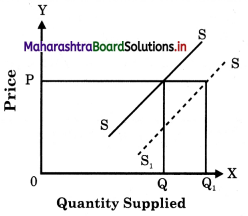At the same price OP, quantity supplied increases from 0Q to 0Q1
Thus, this is a case of increase in supply.Question 5.
Mr. Kriplani produced 2000 bales of cloth during the year 2018-19.
Concept: Total Output.
Explanation : Total Output is the sum total of the commodity produced at a given period of time.
So, total output of Mr. Kriplani is taken as total output for the year 2918-19.

Question 6.
Mr. Kriplani had 200 apples in hand. He bought 500 apples from wholesaler.
Concept: Stock
Explanation : Stock is the total quantity of commodity available for sale with a seller at a particular point of time.
Now in our case, Mr. Kriplani has total stock of 700 apples (200+500) which he can offer for sale at particular price.

Question 7.
Mr. Chaturvedi has 5000 kg rice at his disposal. He offered 2000 kg rice in market at Rs. 25 per kg.
Concept: Stock and Supply
Explanation : Supply means the various quantities of a commodity offered for sale by
producer during a given period of time at a particular price.
In above case, total stock is 5000 kg rice and total supply is 2000 kg rice.

Question 8.
At price Rs. 30 per kg Rameshbhai ( supplied 100 kg of wheat, Bhaveshbhai i supplied 150 kg of wheat and Dharmesh bhai supplied 200 kg of rice. ;
Concept : Individual Supply and Market Supply.
Explanation : Individual supply means quantity offer by a single seller at a given price while market supply means total quantity supplied by all the seller in the market a given price.
In above case, 100kg, 150kg and 200 kg is called as individual supply while 450kg is called as market supply.

Question 9.
A seller has go to village urgently. So, he sells his product at low price even if other seller are selling similar product at higher price. Given economic reason in what way it is related to the law of supply.
This is an exception to the law of supply. As per the law, more is sold at higher price and less at lower price. In this case an individual seller is in need of cash, as he has to go to village urgently, so he has to sell his product at lower price.

(B) Distinguish between:

Question 1.
Output and Supply

 Output Supply 1. Output refers to the total quantity of a commodity that a producer produces with the help of factors of production using a particular technology during a given period of time. (a) Supply refers to the quantity of a commodity which producers are willing to offer for sale at a given price during a given period of time. 2. Output is a function of input. So, O = f (Inputs) (b) Supply is a function of stock. So, S =f (Stock) 3. Output depends upon the level of investment, technique of production, cost of production, availability of factors and natural resources, etc. (c) Supply depends on the stock and price. 4. Output forms the basis of stock. (d) Stock forms the basis of supply.

Question 2.
Individual Supply and Market Supply
Individual Supply:
(a) Individual Supply schedule refers to various quantities of a commodity that an individual seller is willing to sell in the market at different prices during given period of time.
(b) Individual Supply is a narrow concept. It is a part of market_supply.
(c) Schedule: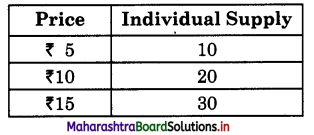(d) As price rises, a seller likes to sell more.

Market Supply:
(a) Market Supply schedule refers to various quantities of a commodity that all sellers are willing to sell in the market at different prices during given period of time.
(b) Market Supply is a broader concept. It is sum total of individual supply.
(c) Schedule: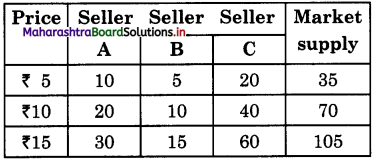(d) As price rises total quantity supplied in the market increases.Question 3.
Individual Supply Curve and Market Supply Curve
Individual Supply Curve:

1. Individual Supply Curve An individual supply curve is a graphical representation of various quantities of a commodity offered for sale by an individual seller at different prices during a given period of time.
2. It is a narrow concept, as it is a part of market supply.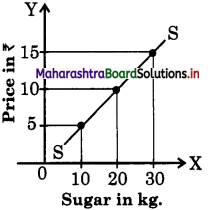3. The above supply curve shows that as price rises quantity supplied increases to 10, 20, 30 kg.

Market Supply Curve:

1. Market supply curve is the graphical representation of the various quantities of a commodity offered for sale by all the sellers at different prices during a given period of time.
2. It is a broader concept, as it sum total of individual supply.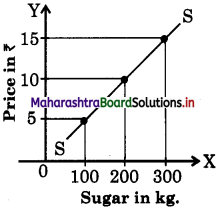3. The above supply curve shows that as price rises, total supply in the market rises to a greater extent to 100, 200, 300 kg.

Question 4.
Extension of Supply and Contraction of Supply.
Extension of Supply:

1. When the supply of a commodity rises only due to increase in the price of that commodity, then it is said to be extension of supply.
2. Extension in supply is shown by an upward movement to right on the same supply curve.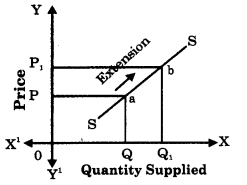3. When price rises from OP to 0P1, Supply extends from 0Q to 0Q1.

Contraction of Supply :

1. When supply of a commodity falls only due to fall in the price of that commodity, then it is said to be contraction of supply.
2. Contraction is shown by the downward movement to left on the same supply curve.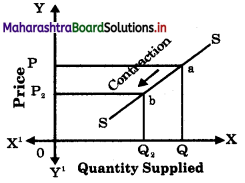3. When prices falls from 0P to 0P2, Supply contracts from 0Q to 0Q2.

Question 5.
Variation in supply and Changes in Supply.
Variation in Supply:

1. Variation in supply refers to expansion and contraction of supply which takes place due to change in price.
2. Expansion means more quantity is supplied at a high price while contraction means less quantity is supplied at a lower price.
3. Variation in supply is caused by change in price.
4. Variation is shown by a upward or downward movement on the same supply curve.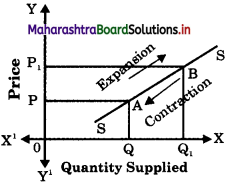Changes in Supply:

1. Changes in supply refers to increase and decrease in supply which takes place due to changes in factors other than price.
2. Increase in supply means more quantity is supplied at same price and decrease means less quantity supplied at the same price.
3. Changes in supply occurs due to change in factors like.
(1) change in cost of production
(2) change in techniques of production
(3) change in government policy.
(4) change in goal of producer.
(5) change in climatic condition etc.
4. Changes in supply is shown by a shift in supply curve to the right or left of the original supply curve.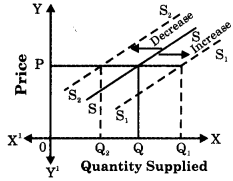Question 6.
Total Cost (TC) and Total Revenue (TR).
Total Cost (TC) :

1. TC is the total expenditure incurred by a firm on the factors of production required for production of goods and services.
2. TC is sum total of Total Fixed Cost (TFC) and Total Variable Cost (TVC). TC=TFC+TVC
3. TC increases with the increase in output.
4. TC depends on factors like cost of raw material, cost of factors like labour, land, capital, technology, cost of advertisement, management, etc.
5. When TC > TR firm faces loss.

Total Revenue (TR) :

1. TR refers to the amount received (income earned) by a firm from the sale of given quantity of a commodity at different price.
2. TR is calculated by multiplying price with the quantity sold. TR = Price X Total Quantity
3. TR increases with increase in sale.
4. Total Revenue (TR) depends on the price of product, demand for the product, etc.
5. When TR > TC firm earns profit.Question 7.
Demand Curve and Supply Curve.
Demand Curve:

1. Demand curve is the graphical representation of a given demand schedule.
2. The demand curve slopes downward from left to right.
3. The demand curve has a negative slope as price and demand are inversely related.
4. The demand curve indicates the consumer’s behaviour in response to change in its price.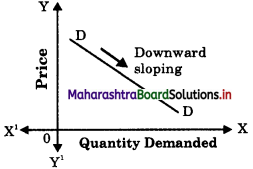Supply Curve:

1. Supply curve is a graphical representation of a given supply schedule.
2. The supply curve slopes upward from left to right.
3. The supply curve has a positive slope as price and supply are directly related.
4. The supply curve indicates the seller’s behaviour in response to change in its price.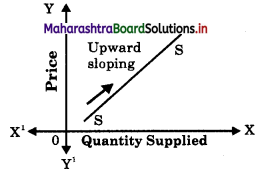Question 8.
Increase in Supply and Decrease in Supply.
Increase in Supply :

1. The supply is said to increase if at the same price more is supplied.
2. Increase in supply is a case of changes in supply.
3. Supply increases due to
(1) fall in cost of production
(2) improvement in transport facility
(3) introduction of modern technology
(4) government subsidies
(5) more imports etc.
4. When there is an increase in supply, the supply curve shifts to the right of original supply curve.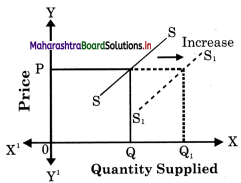Decrease in Supply

1. Decrease in supply occurs when less quantity is supplied at the same price.
2. It is a case of changes in supply.
3. Supply decreases due to –
(1) increase in cost of production
(2) transport strike
(3) outdated technique
(4) heavy taxes imposed by government.
(5) more exports etc.
4. When there is curve shifts to curve.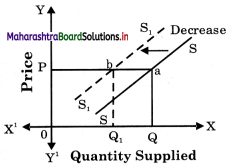Question 9.
Demand and Supply.
Demand:

1. Demand is a desire backed by ability and willingness to pay.
2. Demand is inversely related to price.
3. Demand curve has negative slope.

Supply:

1. Supply means the various quantities offered for sale by a producer at a given price and at a given period of time.
2. Supply is directly related to price.
3. Supply curve has positive slope.

Question 1.
What are the exceptions of the law of supply?
There are some exceptions to the law of s supply. Following are such cases when supply may fall with the rises in price or rise with the fall in price.

(1) Labour supply : Supply of labour in the terms of hours of work is an important exception pointed out by economists. Generally when wages rise, workers work more, but after a certain point if wages continue to rise, supply of labour falls i.e. workers wish to earn more by work in for less hours and supply curve of labour would bend backwards as shown below :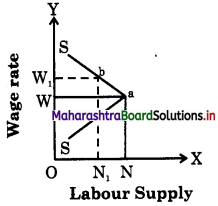In this figure as wage rate rises up to 0W, ;i supply of labor also rises up to ON, but when wage rate rises to 0W1, labour supply falls from ON to 0N1 Hence an exception.

(2) Saving : In case of savings generally it is observed that as the rate of interest rises, savings also rises but some people want to have a fixed regular income by way of interest. They may save less at a higher rate ( of interest and save more at a lower rate of interest. For example : suppose a person is interested in earning a fixed income of ? 800 p.a. then he saves ₹ 10,000/- at 8% rate of interest but when rate of interest increases to 10%, he will save only ₹ 8,000/-.

(3) Future Expectations: If the seller expects a fall in price in future, then he will supply more today even at a low price. But if he expects the prices to rise further in future he will withhold the supply today to supply more in future at a high price.

(4) Need for Cash : When the sellers are in urgent need of liquid cash, then even at a lower price they will offer more goods for sale.

(5) Rare Goods : In case of rare collections such as rare painting, old coins, antique, the law is not applicable as the supply remains fixed. The supply curve is a vertical straight line parallel to Y axis.

(6) Agricultural Goods: Supply of agricultural product is influenced by natural factors like climatic conditions, rainfall etc., which cannot be controlled by man. So in bad weather condition, even at a higher price the supply of agricultural commodities will not increase.Question 2.
Explain in detail labour supply as an exception to the law of supply.
Labour Supply : An interesting exception to the law of supply is provided by the supply curve of labour. In case of supply of labour we come across an unusual phenomenon of the backward bending supply curve. In the beginning, the supply curve of labour slopes upwards as usual from left to right because at higher wage, the units of labour are tempted to offer more hours of work. This continues till the time wage rates have risen sufficiently. When wage rate goes beyond a particular level the worker prefers to substitute work by leisure and cut short the supply of labour. Hence, beyond a certain wage rate the supply curve of labour slopes backwards. This is called the backward bending supply curve of labour and is an exception to the law of supply as shown in the diagram.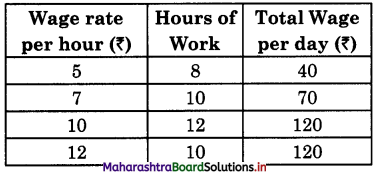In the above schedule, the supply of labour rises from 8 hours to 10 to 12 hours as the wage rate rises from ₹5 per hour to ₹7 to ₹10 per hours. But thereafter when wage rate – becomes ₹12 per hour the supply of labour ; falls to 10 hours. So the supply curve has a backward bend from point ‘A’. Initially, it slopes upwards i.e., SA then A to S1 upto it has a backward slope.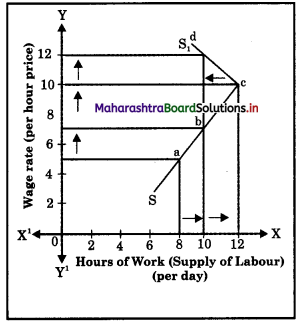Question 3.
Explain variation in supply with the help of diagram.
When quantity supplied of a commodity changes due to change in its price, other factors remaining constant, it is known as variation in supply. Variation in supply can be of two types :
(A) Expansion (or Extension) of Supply
(B) Contraction of Supply

(A) Expansion (or Extension) of Supply : It refers to a rise in the quantity supplied due to an increase in price of a commodity, other factors remaining constant. Extension of supply leads to an upward movement on the same supply curve due to a rise in price as shown in the diagram :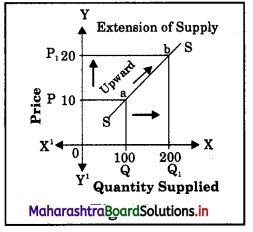In the above diagram, when price increases from P to P1, the quantity supplied rises or expands from Q to Q1 resulting in an upward movement from point A to point B along the same supply curve. It is known as extension of supply.

(B) Contraction of Supply : It refers to fall in quantity supplied due to fall in price, other things remaining constant. Contraction of supply leads to a downward movement on the same supply curve due to a fall in price as shown in the diagram.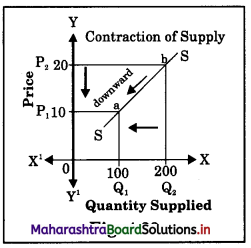In the diagram when price falls from P2 to P1, the quantity supplied falls or contracts from Q2 to Q1 resulting in a downward movement from point B to A along the same supply curve. It is known as contraction of supply.

Question 4.
Explain change in supply with the help of diagram.
OR
Explain increase in supply and decrease in supply.
Changes in supply occurs when the quantity supplied changes due to changes in factors other than price.
Changes in Supply are of two types :
(A) Increase in Supply
(B) Decrease in Supply

(A) Increase in Supply : It means when more quantity is supplied at the same price. Increase in supply occurs due to changes in factors other than change in price. It takes place when –

1. There is decrease in price of inputs
3. Imports are more
4. There is decrease in tax, etc.

Increase in supply is shown by a shift in i the supply curve to the right of the original; supply curve.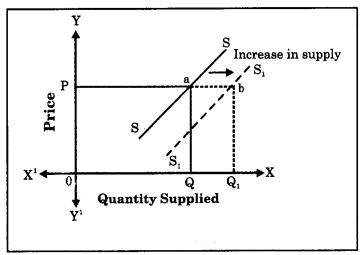In the diagram, at OP price when quantity supplied rises from OQ to OQ1 it indicates) an increase in supply. This is shown by a c shift in supply curve from SS to S1S1 to the right.

(B) Decrease in Supply : It means when less quantity is supplied at the same price.
Decrease in supply occurs due to change in factors other than change in price. It takes place when –

1. there is increase in price of inputs,
2. technological degradation or out dated technology used.
3. exports are more.
4. there is increase in taxes etc.

Decrease in supply is shown by a shift in the supply curve to the left of the original supply curve.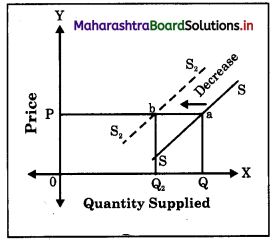At OP price when quantity supplied falls from OQ to OQ2, it indicates a decrease in supply. This is shown by a shift in supply curve from SS to S2S2 to the left.

Question 5.
Explain concept of TC, AC and MC.
Cost of production is an important factor in governing the supply of a product. Following are the three main types of costs :

(A) Total Cost (TC): It is the total expenditure incurred by a fir m on the factors of production required for the production of goods and services. Total cost is the sum of Total Fixed Cost (TFC) and Total Variable Cost (TVC). Total Fixed Cost is the cost incurred on fixed factors of production like land, factory, building, capital, etc. These factors cannot be changed in the short period. They remain constant. Total Variable Cost is the cost incurred on variable factors such as raw – materials, labour, etc. These factors can be varied or changed according to the change in output level. So the variable cost varies. Total Cost = Total Fixed Cost + Total Variable Cost i.e., TC = TFC + TVC
TC increases as the level of output increases.

(B) Average Cost (AC) : Refers to per unit total cost of production. The average cost is obtained by dividing Total Cost of production by the number of units of that commodity produced
i.e. AC = $$\frac{\mathrm{TC}}{\text { Total Output }}$$
Suppose the total cost of producing 100 units of commodity X is ₹ 1000. Then the average cost is
AC = $$\frac{\mathrm{TC}}{\text { Total Output }}=\frac{1000}{100}$$ = 10
∴ AC = ₹ 10

(C) Marginal Cost (MC) : It is net addition made to the total cost (TC) by producing one ( more unit of output.
Suppose the total cost of producing 2 units is ₹ 200 and TC of producing 3 unit is ₹ 240.
MCn = TCn – TCn-1 = ₹ 240 – ₹ 200 = ₹ 40
Where,
n = Number of unit produced ?
MCn = Marginal Cost of the nth unit ?
TCn = Total Cost of n unitQuestion 6.
Explain the concepts of TR, AR, and MR.
Revenue refers to the income earned by a firm from the sale of given quantity of a commodity in the market at different prices. Following are the three main types.

(A) Total Revenue (TR) : Total Revenue (Income) refers to total receipts of the firm from its sales of commodity. It is obtained i by multiplying the price per unit of the commodity with the total number of units!; of commodity sold to the consumers. Thus, Total Revenue = Price per unit x Total Number of units of commodity sold.
TR = Price x Quantity Sold

Example : If the firm sells 10 units of) a commodity at ₹100 per unit then total?
revenue will be TR = 100 x 10. TR = ₹1000

(B) Average Revenue (AR): Average Revenue refers to the revenue (income) per unit of the commodity sold. It can be easily calculated by dividing Total Revenue (TR) by the number of units sold to the consumers. Thus, Average Revenue = Total Revenue + Number of units sold.
i.e., AR = $$\frac{\mathrm{TC}}{\text { Total Quantity Sold }}$$

For example : If the Total Revenue from? the sale of 10 units of commodity is ₹ 1000; then Average Revenue will be
AR = $$\frac{\mathrm{TR}}{\mathrm{Q}}=\frac{1000}{10}$$ = ₹100

(C) Marginal Revenue (MR) : Marginal Revenue is the net addition made to TR by selling an additional unit of the commodity. In other words, marginal revenue is the addition made to the total revenue by selling one more unit of a commodity.

Example : If the total revenue from the sale of 10 units is ?1000 and that from the sale of 11th unit the total revenue is 1020 then the MR of 11th unit will be
MRn = TRn – TRn-1 = 1020 – 1000 = 20
Or we can also calculate Marginal Revenue as –
MR = $$\frac{\text { Change in Total Revenue }}{\text { Change in total number of units sold }}$$
MR = $$\frac{\Delta \mathrm{TR}}{\Delta \mathrm{T} Q}=\frac{20}{1}$$ = 20
MR = 20

Question 7.
What are the assumptions to the law of supply?
Assumptions of the Law of Supply : The law of supply is conditional. It is based on certain assumptions. They are as follows:

1. No change in Cost of Production: The law will hold true only if the cost of production remains constant.
2. No change in Technique of Production : It is assumed that there is no change in the method of production. If there is any improvement in the technique of production then supply will increase at the same price.
3. 3. No change in Weather condition : Changes in weather and climatic condition would affect supply especially of agricultural products, so it is assumed to remain constant.
4. No change in Government Policies : Any change in government policies will affect the supply, so it is assumed that there is no change in government tax policies, subsidies or industrial policy.
5. No Speculations about Future Prices : It is assumed that the producers do not speculate about future changes in price.
6. No change in Transport Cost : It is assumed that there is no change in transport facility and transport cost.
7. Prices of Other Goods : It is assumed that the prices of competitive goods remain constant. If the price of competitive goods rises then it is quite likely that producer may transfer his resource to the production of those goods, whose price has arisen.
8. No change in the quantity of goods kept for Self – consumption : If sellers keep more goods for their personal use then supply cannot be increased even at a high price.
9. Constant Scale of Production : It is assumed that the scale of production remains constant during the given period of time.

4. State with resons whether you agree or disagree with the following statements.

Question 1.
Supply is directly related to price.
Yes, I agree with this statement.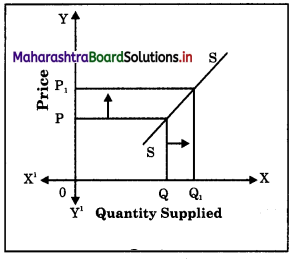• According to the Law of Supply, other things being equal, the supply varies directly with its price.
• More quantity is offered for sale at a higher price and less is offered for sale at a lower price.
• So supply is directly related to its price.
• In order to earn a reasonable profit, the sellers tries to sells more at a higher price and less at a lower price.
So the supply curve slopes upwards. It has a positive slope.
• Thus, the supply is directly related to price.

Question 2.
Stock can exceed supply.
OR
Supply cannot exceed stock.
Yes, I agree with this statement.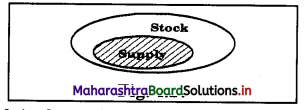• Stock is the entire quantity of a commodity, which is in the custody of the seller.
• Supply refers to that part of stock of a commodity which is offered for sale at prevailing market price during a period of time.
• Thus supply is a part of stock. c
• Stock is the potential supply. It is a fund or reservoir from which supply flows.
• Stock determines the ability of seller to supply.
Thus, stock exceeds supply.

Question 3.
When price rises supply expands.
Yes, I agree with this statement.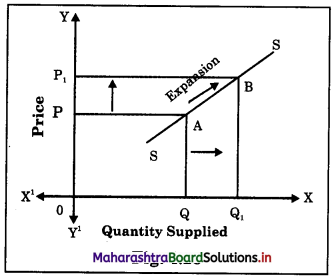• According to the Law of Supply “Other things remaining constant more is supplied < at a higher price and less at a lower price.”
• The rise in price causes an expansion in supply because (i) the existing sellers will supply more than before in order to get larger profit and (ii) new suppliers with high reservation price will also now enter S the market.
• So supply expands when price rises.

Question 4.
The supply curve has a positive slope..
OR
The supply curve slopes upwards from left to right.
OR
The relationship between price and supply is positive.
OR
There is direct relation between price and quantity supplied.
Yes, I agree with this statement.
OR The supply curve slopes downward from left to right.
No, I do not agree with this statement.

• According to the Law of Supply “Other things remaining constant supply varies directly with price i.e., more quantity will be offered for sale at a higher price and less at a lower price. So there is a positive relationship between price and supply.”
S = f(P)
• The supply curve which is a graphical representation of supply schedule slopes upward from left to right indicating a positive slope.
• In order to earn reasonable profit, the sellers try to sell more at higher prices and less at lower prices.
• The sellers earn more profit when market price is greater than their reservation price.
• Thus, the above statement is true.

Question 5.
Agricultural products are exceptions to the law of supply.
Yes, I agree with this statement.

• The law of supply states that the supply of a commodity varies directly with its price.
• But in case of agricultural goods supply does not vary directly with price.
• Agricultural product being perishable in nature cannot be stored for long time. The sellers cannot wait for price to rise. They have to sell off the whole stock even at a low price. Here supply coincides with stock.
• Also agricultural production depends on unforeseen changes in weather. So even at higher price supply cannot be increased during drought or flood situation.
• So, the above statement is true.

Question 6.
Due to speedy transport supply falls.
No, I do not agree with this statement.

• Transport facilities are one of the important determinants of supply.
• Modern speedy transport facilities in widening r the market.
• It increases the mobility of goods and helps in creating place utility for goods and services.
• Speedy transport helps in domestic as well; as international trade of goods and services.
• Thus, the above statement is false.

Question 7.
Reservation price is very low in respect of perishable commodities.
Yes, I agree with this statement. ;

• Reservation price is sellers minimum price, below which the seller will not supply any unit of the commodity.
• Whether the sellers reservation price will be high or low depends upon number of factors. One of them is the durability of the commodity.
• If the commodity is durable like TV set, car etc., then sellers reservation price will be high.
• But if the commodity is perishable like fish, flowers, vegetables, milk etc., then reservation price will be low.
• As perishable commodities cannot be stored for long, it can become stale and useless.
Therefore, sellers are forced to clear stock as early as possible.
So the reservation price is low.

Question 8.
Supply depends on many factors.
OR
Market Supply depends on many factors.
Yes, I agree with this statement.
OR
Price is the only determinant of Supply.
No, I do not agree with this statement.
Supply depends on many factors. Price is the main factor. There is a direct relationship between price and supply. Besides price there are many other factors such as

• Cost of production and supply are indirectly related. Higher the cost, lesser will be the supply.
• State of technology i.e., advanced technology increases supply.
• Government policy like subsidies encourage production and increases supply, whereas heavy taxation decreases supply.
• Natural conditions like favourable weather condition increases supply.
• Exports decreases supply and imports increases supply.
Better infrastructure facility increases supply.
• Nature of market like competitive market increases supply.
Thus, the above statement is true.

Question 9.
Total cost includes Total Fixed Cost and Total Variable Cost.
Yes, I agree with this statement.

• Total cost is the total cost incurred by a firm on the factors of production required for production of goods and services. Total cost is the sum total of fixed cost and variable cost.
• Total fixed cost includes cost incurred on fixed factors like factory building, plant size, machinery etc. Fixed cost remains fixed in the short run but changes in the long period. Total variable cost includes cost on variable factors like raw materials, fuel, labour etc., which changes according to the output in the short period also. So while calculating Total Cost we include Total Fixed Cost (TFC) and Total Variable Cost (TVC). So, TC = TFC + TVC. Thus, the above statement is true.

Question 10.
Supply of perishable goods is equal to its production.
OR
There is no difference between stock and supply of perishable goods.
Yes, I agree with this statement.

• The stock of perishable goods like vegetables, flowers, fruits, milk, etc., is equal to supply.
• This is because perishable goods cannot be stored for long time. They become stale.
• They need to be sold within a short period of time irrespective of the price.
• Also the cost of storage is high.
• So, the above statement is true.

Question 11.
The supply curve of labour is backward bending.
Yes, I agree with this statement.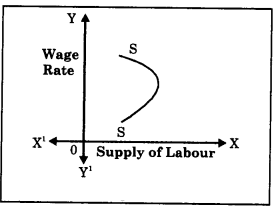• When the wage rate in the industry rises, the supply of labour (i.e., hours of work) rises upto a certain limit.
• Beyond certain limit with further rise in wages, supply of labour tends to fall.
• This is because the labour prefers leisure to work i.e., he substitutes work with leisure,
• With the increased wage rate he is able to satisfy his needs.
• So the supply curve instead of rising upwards
starts sloping backward as he reduces the hours of work.

Question 12.
AR is always more than TR.
No, I do not agree with this statement.

• TR or Total Revenue refers to total income earned by a firm from the sale of a given quantity of a commodity in the market of different price. It is derived by Price x l Quantity sold.
• AR or Average Revenue refers to the income earned per unit of a commodity sold. It is derived by TR Quantity sold.
• Hence, TR will always be greater than AR.
Hence, the above statement is false.Question 13.
Demand and Supply are opposite forces.
Yes, I agree with this statement.
Both demand and supply are opposite forces.

• Demand explains the consumer’s behaviour with respect to change in price of a commodity. Supply explains the producer’s behaviour with respect to change in price of a commodity.
• Demand is inversely related to price of a commodity i.e., when price of a commodity rises the demand for it falls and when price falls the demand rises. Supply is directly related to the price i.e., when price rises, supply expands and when price falls, supply falls or contracts.
• The demand curve slopes downwards. It has a negative slope. The supply curve slopes upwards from left to right. It has a positive slope. Both opposite forces together determine the equilibrium price.
• A consumer aims at maximum satisfaction with his limited income. So he demands more at a lower price and less at a higher price. Whereas a producer aims at maximum profit so he supplies more at a higher price and less at a lower price.
• Thus, the above statement is true.

Question 14.
There is difference between stock and supply.
Yes, I agree with this statement.
OR
There is no difference between stock and supply.
No, I do not agree with this statement.

 Stock Supply (a) Stock refers to the total quantity of commodity available with producer for sale. (a)Supply is that part of stock which the seller is willing to offer for sale at a given price. (b) It is outcome of production. If production increases, stock will also increase. (b) It is outcome of stock. Stock is the basis of supply. (c) It is a fund or reservoir and a static concept (inelastic). (c) It is a flow concept. It changes according to change in price (elastic). (d) It can exceed supply. (d) It cannot exceed stock.

Question 15.
There are no exceptions to law of supply.
No, I do not agree with this statement.
There are some exceptions to the law of s supply. Following are such cases when supply may fall with the rises in price or rise with the fall in price.

(1) Labour supply : Supply of labour in the terms of hours of work is an important exception pointed out by economists. Generally when wages rise, workers work more, but after a certain point if wages continue to rise, supply of labour falls i.e. workers wish to earn more by work in for less hours and supply curve of labour would bend backwards as shown below :In this figure as wage rate rises up to 0W, ;i supply of labor also rises up to ON, but when wage rate rises to 0W1, labour supply falls from ON to 0N1 Hence an exception.

(2) Saving : In case of savings generally it is observed that as the rate of interest rises, savings also rises but some people want to have a fixed regular income by way of interest. They may save less at a higher rate ( of interest and save more at a lower rate of interest. For example : suppose a person is interested in earning a fixed income of ? 800 p.a. then he saves ₹ 10,000/- at 8% rate of interest but when rate of interest increases to 10%, he will save only ₹ 8,000/-.

(3) Future Expectations: If the seller expects a fall in price in future, then he will supply more today even at a low price. But if he expects the prices to rise further in future he will withhold the supply today to supply more in future at a high price.

(4) Need for Cash : When the sellers are in urgent need of liquid cash, then even at a lower price they will offer more goods for sale.

(5) Rare Goods : In case of rare collections such as rare painting, old coins, antique, the law is not applicable as the supply remains fixed. The supply curve is a vertical straight line parallel to Y axis.

(6) Agricultural Goods: Supply of agricultural product is influenced by natural factors like climatic conditions, rainfall etc., which cannot be controlled by man. So in bad weather condition, even at a higher price the supply of agricultural commodities will not increase.

Question 16.
Price is the only determinant of supply.
No, I do not agree with this statement.

1. Cost of Production : Changes in the price of factors of production like rent, wages, interest affects the cost of production. When cost of production increases, supply decreases.
2. Price of Other Goods : The supply of a given commodity depends on the price of other commodity. E.g. if the price of wheat rises and that of rice remains the constant, then the producer will think of producing more of wheat. This will affect the supply of rice.
3. price of the Commodity : Price is an important factor influencing the supply. More is supplied at a higher price and less at a lower price. So price and supply are 5 directly related.
4. Climatic Conditions : The supply of commodity is also influenced by the forces
5. Government Policy : Government policies like taxation, subsidies, industrial policies etc., may encourage or discourage production and supply. A tax on the commodity will raise the cost of production and reduce the supply while a subsidy on the other hand will provide an incentive to increase production and supply.
6. Exports and Imports : When the
government resort to imports, supply expands, at the same time heavy exports would reduce the supply in the domestic market.
7. Nature of Market : In a competitive market, the supply would be more but in a monopoly market the seller may create artificial scarcity to raise the price.
8. Future Expectation : If future trends indicate a rise in price, the supply decreases at present. On the other hand if the sellers expect the future price to fall, supply would increase in the current period.
9. Technique of Production : Improvement in the technique of production will lead to increase in supply. Application of advanced technology enables the producer to produce goods on large scale at a lower cost and lesser price.
10. Infrastructure Facility : If means of transport and communication are well developed, the extent of market would be wide. i.e. supply will increase.
11. Natural and Man-made Calamities : Natural calamities like earthquake, cyclone, flood etc., will affect the supply in the market. Even man-made calamities like a bomb-blast, affects supply. Even a strike call can affect supply in the market.5. Study the following table / figures and answer the questions :

Question 1.
TFC (Total Fixed Cost) of a commodity ‘x’ of a firm is ₹ 20 Calculate (a) TVC and (b) TC from the following data.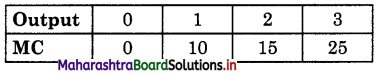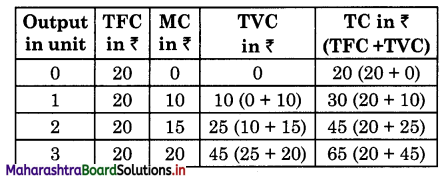Question 2.
Find TR of a firm if it sells 500 units of £ commodity ‘X? at 25 ₹ per unit.
TR is the revenue derived by a firm by multiplying total units sold by its price.
Hence, TR = P x Q
= 25 x 500
= 12, 500₹

Question 3.
Calculate MC at each level of output of the following data.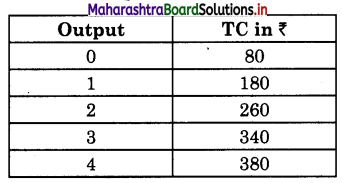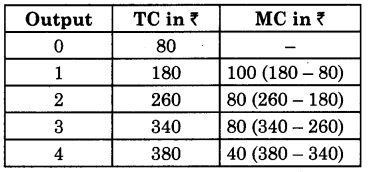Question 4.
Workout : Price of oranges is ₹ 200 per dozen. During festival time at the same price 2 dozen, oranges are offered for sale. On the basis of this answer the following

1. Draw the figure for this.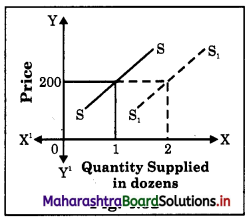2. Is it the case of variation or change in supply. Give reason.
It is a case of change in supply, as here the price remains the same at ? 200, while quantity increases from 1 dozen to 2 dozen. So, here supply changes due to festival season and due to change in price.Question 5.
Demand in world market for Indian antique goods have increased but supply of these are fixed even though buyers are ready to pay high price for it. On the basis of this answer the following :

1. Draw figure for this.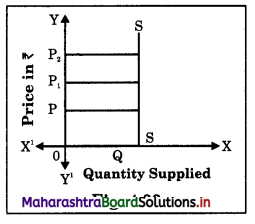Question 6.
Does this justify the law of supply? Give reason for your answer.
No, it does not justify the law of supply. As per law of supply, as the price increases, supply of a commodity should increase. But in above case even though the price is increasing supply remains the same. So it is an exception to the law of supply.

Question 1.
State and explain the law of supply. What are its assumptions?
Law of Supply :
(A) Introduction : The law of supply was introduced by Dr. Alfred Marshall in his book “Principles of Economics” published in 1890. The law establishes a functional relationship between the price of a commodity and quantity supplied of that commodity. It explains the general tendency of the sellers in offering more goods for sale at a higher price than at a lower price.

(B) Statement of the Law : According to Prof. Alfred Marshall “Other things remaining constant, the higher the price of the commodity, greater is the quantity supplied and lower the price of the commodity, smaller is the quantity supplied.”In other words, quantity supplied of a commodity varies directly with price i.e., with a fall in price supply contract and with a rise in price supply expands.
S = f (P) [S = Supply, P = Price, f = Function of
The law can be better understood with the help of a market supply schedule and market supply curve.

(C) Market Supply Schedule : Market supply schedule is a tabular representation of various quantities of a commodity offered for sale by all the sellers in the market at different prices during a given period of time. The schedule is a hypothetical one except one price rest are imaginary prices.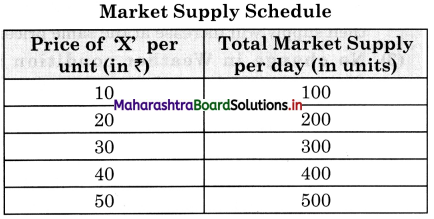The above schedule clearly shows that sellers in general want to sell more at high prices and less at low price. E.g., at a low price of ₹10 per unit the seller supplies only 100 units per day and at high price of ₹ 50 the supply rises to 500 units of ‘X’ per day.

(D) Market Supply Curve : It is graphical representation of the above market supply schedule. Price is measured on ‘Y’ axis and quantity supplied on ‘X’ axis and above schedule is plotted. We derive a supply curve SS. ’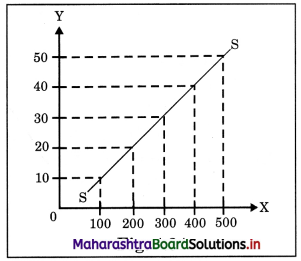In the diagram, supply curve SS is a linear supply curve (straight – line). It slopes upwards from left to right. It has a positive slope indicating direct relationship between price and quantity supplied.

(E) Assumptions of the Law of Supply : The law of supply is conditional. It is based on certain assumptions. They are as follows:

• No change in Cost of Production: The law will hold true only if the cost of production remains constant.
• No change in Technique of Production : It is assumed that there is no change in the method of production. If there is any improvement in the technique of production then supply will increase at the same price.
• No change in Weather condition : Changes in weather and climatic condition would affect supply especially of agricultural products, so it is assumed to remain constant.
• No change in Government Policies : Any change in government policies will affect the supply, so it is assumed that there is no change in government tax policies, subsidies or industrial policy.
• No Speculations about Future Prices : It is assumed that the producers do not speculate about future changes in price.
• No change in Transport Cost : It is assumed that there is no change in transport facility and transport cost.
• Prices of Other Goods : It is assumed that the prices of competitive goods remain constant. If the price of competitive goods rises then it is quite likely that producer may transfer his resource to the production of those goods, whose price has arisen.
• change in the quantity of goods kept for Self – consumption : If sellers keep more goods for their personal use then supply cannot be increased even at a high price.
• Constant Scale of Production : It is assumed that the scale of production remains constant during the given period of time.

Question 2.
State and explain the law of supply with exceptions.
Law of Supply :
(A) Introduction : The law of supply was introduced by Dr. Alfred Marshall in his book “Principles of Economics” published in 1890. The law establishes a functional relationship between the price of a commodity and quantity supplied of that commodity. It explains the general tendency of the sellers in offering more goods for sale at a higher price than at a lower price.

(B) Statement of the Law : According to Prof. Alfred Marshall “Other things remaining constant, the higher the price of the commodity, greater is the quantity supplied and lower the price of the commodity, smaller is the quantity supplied.”In other words, quantity supplied of a commodity varies directly with price i.e., with a fall in price supply contract and with a rise in price supply expands.
S = f (P) [S = Supply, P = Price, f = Function of]
The law can be better understood with the help of a market supply schedule and market supply curve.

(C) Market Supply Schedule : Market supply schedule is a tabular representation of various quantities of a commodity offered for sale by all the sellers in the market at different prices during a given period of time. The schedule is a hypothetical one except one price rest are imaginary prices.The above schedule clearly shows that sellers in general want to sell more at high prices and less at low price. E.g., at a low price of Rs. 10 per unit the seller supplies only 100 units per day and at high price of Rs. 50 the supply rises to 500 units of ‘X’ per day.

(D) Market Supply Curve : It is graphical representation of the above market supply schedule. Price is measured on ‘Y’ axis and quantity supplied on ‘X’ axis and above schedule is plotted. We derive a supply curve SS. ’In the diagram, supply curve SS is a linear supply curve (straight – line). It slopes upwards from left to right. It has a positive slope indicating direct relationship between price and quantity supplied.

There are some exceptions to the law of s supply. Following are such cases when supply may fall with the rises in price or rise with the fall in price.

(1) Labour supply : Supply of labour in the terms of hours of work is an important exception pointed out by economists. Generally when wages rise, workers work more, but after a certain point if wages continue to rise, supply of labour falls i.e. workers wish to earn more by work in for less hours and supply curve of labour would bend backwards as shown below :In this figure as wage rate rises up to 0W, ;i supply of labor also rises up to ON, but when wage rate rises to 0W1, labour supply falls from ON to 0N1 Hence an exception.

(2) Saving : In case of savings generally it is observed that as the rate of interest rises, savings also rises but some people want to have a fixed regular income by way of interest. They may save less at a higher rate ( of interest and save more at a lower rate of interest. For example : suppose a person is interested in earning a fixed income of ? 800 p.a. then he saves ₹ 10,000/- at 8% rate of interest but when rate of interest increases to 10%, he will save only ₹ 8,000/-.

(3) Future Expectations: If the seller expects a fall in price in future, then he will supply more today even at a low price. But if he expects the prices to rise further in future he will withhold the supply today to supply more in future at a high price.

(4) Need for Cash : When the sellers are in urgent need of liquid cash, then even at a lower price they will offer more goods for sale.

(5) Rare Goods : In case of rare collections such as rare painting, old coins, antique, the law is not applicable as the supply remains fixed. The supply curve is a vertical straight line parallel to Y axis.

(6) Agricultural Goods: Supply of agricultural product is influenced by natural factors like climatic conditions, rainfall etc., which cannot be controlled by man. So in bad weather condition, even at a higher price the supply of agricultural commodities will not increase.Question 3.
What is meant by Supply? State its determinants.
Law of Supply :
(A) Introduction : The law of supply was introduced by Dr. Alfred Marshall in his book “Principles of Economics” published in 1890. The law establishes a functional relationship between the price of a commodity and quantity supplied of that commodity. It explains the general tendency of the sellers in offering more goods for sale at a higher price than at a lower price.

(B) Statement of the Law : According to Prof. Alfred Marshall “Other things remaining constant, the higher the price of the commodity, greater is the quantity supplied and lower the price of the commodity, smaller is the quantity supplied.”In other words, quantity supplied of a commodity varies directly with price i.e., with a fall in price supply contract and with a rise in price supply expands.
S = f (P) [S = Supply, P = Price, f = Function of]
The law can be better understood with the help of a market supply schedule and market supply curve.

(C) Market Supply Schedule : Market supply schedule is a tabular representation of various quantities of a commodity offered for sale by all the sellers in the market at different prices during a given period of time. The schedule is a hypothetical one except one price rest are imaginary prices.The above schedule clearly shows that sellers in general want to sell more at high prices and less at low price. E.g., at a low price of Rs. 10 per unit the seller supplies only 100 units per day and at high price of Rs. 50 the supply rises to 500 units of ‘X’ per day.

(D) Market Supply Curve : It is graphical representation of the above market supply schedule. Price is measured on ‘Y’ axis and quantity supplied on ‘X’ axis and above schedule is plotted. We derive a supply curve SS. ’In the diagram, supply curve SS is a linear supply curve (straight – line). It slopes upwards from left to right. It has a positive slope indicating direct relationship between price and quantity supplied.

1. Cost of Production : Changes in the price of factors of production like rent, wages, interest affects the cost of production. When cost of production increases, supply decreases.
2. Price of Other Goods : The supply of a given commodity depends on the price of other commodity. E.g. if the price of wheat rises and that of rice remains the constant, then the producer will think of producing J more of wheat. This will affect the supply of rice.
3. price of the Commodity : Price is an important factor influencing the supply. More is supplied at a higher price and less at a lower price. So price and supply are 5 directly related.
4. Climatic Conditions : The supply of commodity is also influenced by the forces
5. Government Policy : Government policies like taxation, subsidies, industrial policies etc., may encourage or discourage production and supply. A tax on the commodity will raise the cost of production and reduce the supply while a subsidy on the other hand will provide an incentive to increase production and supply.
6. Exports and Imports : When the
government resort to imports, supply expands, at the same time heavy exports would reduce the supply in the domestic market.
7. Nature of Market : In a competitive market, the supply would be more but in a monopoly market the seller may create artificial scarcity to raise the price.
8. Future Expectation : If future trends indicate a rise in price, the supply decreases at present. On the other hand if the sellers expect the future price to fall, supply would increase in the current period.
9. Technique of Production : Improvement in the technique of production will lead to increase in supply. Application of advanced technology enables the producer to produce goods on large scale at a lower cost and lesser price.
10. Infrastructure Facility : If means of transport and communication are well developed, the extent of market would be wide. i.e. supply will increase.
11. Natural and Man-made Calamities : Natural calamities like earthquake, cyclone, flood etc., will affect the supply in the market. Even man-made calamities like a bomb-blast, affects supply. Even a strike call can affect supply in the market.